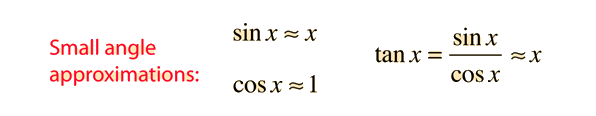# Trig Function Series

Trigonometric functions can be expanded in power series, which facilitates approximations of the functions in extreme cases. The angle x must be in radians.### Applications

Index

Trigonometry

 HyperPhysics*****HyperMath*****Calculus R Nave
Go Back

# Applications of Trig Series

One of the most important applications of trigonometric series is for situations involving very small angles. For such angles, the trigonmetric functions can be approximated by the first term in their series. This gives the useful small angle approximations:Examples of the use of the small angle approximation are in the calculation of the period of a simple pendulum, and the calculation of the intensity minima in single slit diffraction. This approximation is used in most of the common expressions of geometrical optics which are built on the concept of surface power for lenses.

This approximation sin x = x reaches a 1% error at about 14 degrees.

Index

Trigonometry

 HyperPhysics*****HyperMath*****Calculus R Nave
Go Back